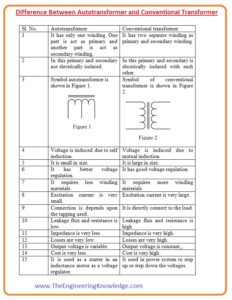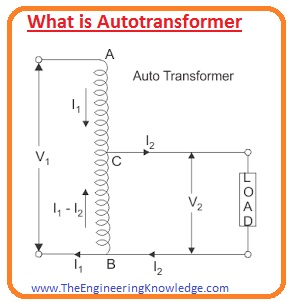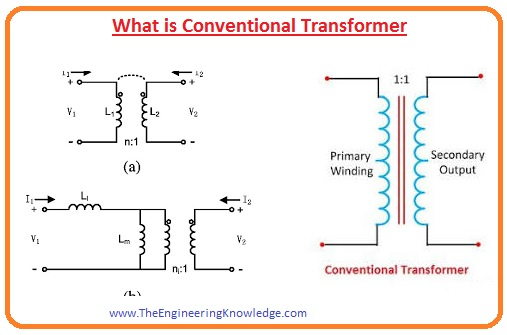Hello, friends, I hope you all are doing great. In today’s tutorial, we will discuss the Difference Between Autotransformer and Conventional Transformer. The basic difference between autotransformer is that in autotransformer one winding is used while in conventional transformer 2 different windings are used.

In today’s post we will have a detailed look at both autotransformer and conventional transformer. We will compare them to find their differences. So let’s get started with Difference Between Autotransformer and Conventional Transformer.

#### Difference Between Autotransformer and Conventional Transformer

Autotransformer

• The type of transformer in which one winding is used and its first part act as primary and second part as secondary.
• In this transformer, only a single winding is used.
• The primary and secondary winding of this transformer is not electrically insulated.
• Its operation is based on the phenomena of self-induction.
• Its physical structure is less than the conventional transformer.
• In this transformer half part of power is transferred through transformation and half through the direct electrical connection.
• Its voltage regulation is better than the conventional transformer.
• It needed less winding conductors than a conventional transformer.
• The primary and secondary circuits of this transformer are linked through magnetically.
• Its connection relies on tapping.
• Its starting current reduces.
• It has less excitation current.
• It is less expensive than a conventional transformer.
• Its efficiency is larger than the conventional transformer.
• the leakage flux and value resistance in this transformer is less than the autotransformer.
• The impedance of this transformer is less than the conventional transformer.
• The value of the output voltage can be varied.
• Its common applications are starter in an induction motor, as a voltage regulator.

Conventional Transformer

• The transformer is a static electrical device that changes electrical energy from one level to other without varying the frequency.
• There are 2 windings is used in this transformer first is the primary and the second one is secondary.
• There is electrical insulation occurs between primary and secondary windings.
• Its operation is based on the phenomena of mutual induction.
• The physical structure of autotransformer is larger than the autotransformer.
• In this transformer power is only transferred through transformation.
• It provides good voltage regulation.
• It needed more conductor for windings than the autotransformer.
• The linking of both windings is electrically and magnetically.
• It is linked directly with the load.
• It needed a large excitation current than the autotransformer.
• Its price is large than the autotransformer.
• Its efficiency is less than the autotransformer.
• The leakage flux and value of resistance for this transformer is high.
• Its impedance value is larger than the autotransformer.
• The occurrence of power losses is large in this transformer.
• Its output voltage has constant values.
• It used in the power system to change the voltage level.#### What is Autotransformer

• The transformer uses single winding and it divided into the 2 parts that act as primary and secondary winding.
• The primary and secondary windings are linked magnetically and electrically.
• If the voltage at secondary winding is larger than the primary winding this transformer is called step up and if the voltage at secondary is less than primary than called step down.
• The price of the autotransformer is less and provides better regulation and has less power losses.
• The drawback of this transformer is that there is no insulation exits between primary and secondary windings/
• So if voltage given at the primary is high value than this voltage will also exit across the secondary so it can be harmful for load and operator.#### What is Conventional Transformer

• The conventionals is an electrical device that used to transformation of electrical energy from primary to secondary winding without varying frequency.
• Its operation is based on the phenomena of electromagnetic induction that say EMF is generated in the circuitry with the change in field in that circuit.
• In this transformer windings have insulation for electrically and connected in a magnetic way.
• There 2 windings is used in this transformer primary and secondary winding. At primary winding, input is given and at secondary winding, load is linked.
• If the voltage at primary is less than it called to step up and if the voltage at primary is large called to step down transformer.
• Such transformer whose voltage at primary and secondary are same called one to one transformer.#### Comparison between Autotransformer and Conventional Transformer

• In autotransformer one winding is used that divided into two parts but in the conventional transformer primary and secondary windings are different.
• The operation of the autotransformer is based on self-induction and conventional transformer is based on mutual induction.
• he auto-transformer is smaller in size, whereas the conventional transformer is larger in size.
• the conventional transformer is less economical than the autotransformer.
• The transfer of power occur in autotransformer through the transformation and in conventional transformer power transferred through mutual induction.
• The voltage regulation of autotransformer is better than the conventional transformer.
• Due to the use of single winding autotransformers needed only less conductor than the conventional transformer.
• The primary and secondary windings of autotransformer have no electrical insulation while conventional transformer has electrical insulation.
• The starting current required for autotransformer is less than the real current and the starting current of the conventional transformer is 1/3 of the actual current.
• The efficiency of the autotransformer is larger than the conventional transformer.
• due to single winding the leakage flux in autotransformer is less than the conventional transformer.
• The impedance of a conventional transformer is larger than the autotransformer.
• The price of a conventional transformer is high than the autotransformer.
• The power loss in a conventional transformer is large than the autotransformer.

So it is a detailed post about the difference between autotransformer and conventional transformer if you have any query ask in comments. Thanks for reading. Have a good day.